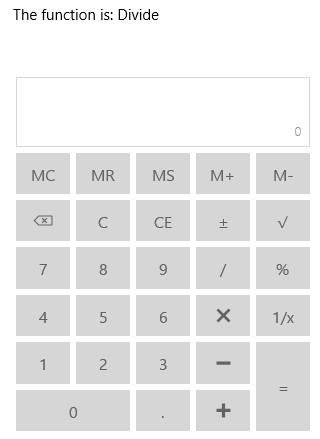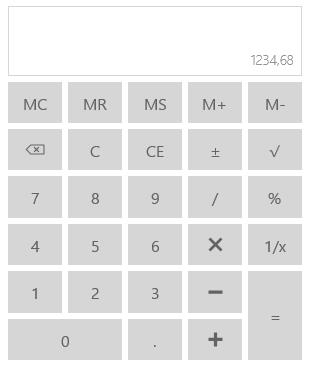# Utilities in UWP Calculator (SfCalculator)

10 May 20212 minutes to read

Several utility functions are available in `SfCalculator` control.

## Identifying the Operation

This method is used to identify the mathematical operation based on the input string.

``````<StackPanel>

<TextBlock x:Name="functionText"/>

<input:SfCalculator x:Name="calculator"/>

</StackPanel>``````
``````CalculatorFunctions function = calculator.CheckFunction("/");

functionText.Text = "The function is: " + function.ToString();``````
``````Dim [function] As CalculatorFunctions = calculator.CheckFunction("/")

functionText.Text = "The function is: " & [function].ToString()``````## Clear the Expression

`Clear` function is used to clear both the expression and value in `SfCalculator`.

``calculator.Clear();``
``calculator.Clear()``

## Clear the Value

`ClearEntry` function is used to clear the entered/computed value in `SfCalculator`.

``calculator.ClearEntry();``
``calculator.ClearEntry()``

## Clear and Reset the Expression

`Reset` function is used to clear and reset the expression.

``calculator.Reset();``
``calculator.Reset()``

## Removing Trailing Zeros

`TrailingZeros` method is used to remove the trailing zeros at the end of value.

``calculator.TrailingZeros();``
``calculator.TrailingZeros()``

## Change Separators based on Culture

`SfCalculator` has Culture support that allows to have decimal separator symbols based on the culture.

``<input:SfCalculator x:Name="calculator"/>``
``calculator.Culture = new System.Globalization.CultureInfo("de-DE");``
``calculator.Culture = New System.Globalization.CultureInfo("de-DE")``